北京大学 985 双一流 211

Peking University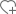关注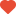已关注•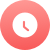1898

•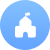公立

•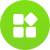综合

•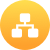教育部

•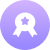本科

•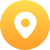北京-海淀区

•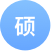330个

•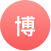302个

院校分数线

多方向招生特色专业

• 经济学
• 西班牙语
• 非通用语种群(印地语、乌尔都语、孟加拉语、梵文、巴利文5个语种)
• 地质学
• 核技术
• 临床医学(与北京大学第一医院结合)
• 口腔医学
• 法学
• 阿拉伯语
• 非通用语种群(蒙古语、菲律宾语、泰国语、波斯语、西伯莱语等12个语种)
• 微电子学
• 软件工程(设5个专业方向)
• 临床医学(与北京大学人民医院结合)
• 哲学
• 化学
• 药学
• 保险
• 信息与计算科学
• 生物科学
• 智能科学与技术
• 城市管理
• 汉语言文学
• 考古学
• 世界历史
• 预防医学
• 国际政治
• 地球物理学
• 地理科学
• 理论与应用力学
• 新闻学
• 环境科学
• 金融学
• 电子信息科学与技术
• 能源与资源工程
• 环境工程院系/专业

• 物理学（本）
• 天文学（本）
• 大气科学（本）
• 核物理（本）

• 应用心理学（本）
• 基础心理学（本）

• 历史学（本）

• 化学生物学系（本）
• 应用化学系（本）

• 金融数学系（本）
• 概率统计系（本）
• 数学系（本）
• 信息科学系（本）
• 科学与工程计算系（本）

• 动物生理学（本）
• 植物生理学（本）
• 微生物学（本）
• 生物物理学（本）
• 生态学（本）
• 环境生物学（本）
• 生物技术（本）
• 细胞生物学（本）
• 动物遗传学（本）
• 生物化学（本）

• 地质学（本）
• 地理信息科学（本）
• 地球物理学（本）
• 地球化学（本）
• 空间科学与技术（本）

• 材料科学与工程（本）
• 生物医学工程（本）
• 能源与资源工程（本）
• 理论与应用力学（本）
• 航空航天工程（本）
• 工程结构分析（本）

• 生态学（本）
• 自然地理与资源环境（本）
• 城乡规划（本）
• 人文地理与城乡规划（本）
• 环境科学（本）

• 环境管理系（本）
• 环境科学系（本）
• 环境工程系（本）

• 电子信息科学与技术（本）
• 微电子学（本）
• 智能科学与技术（本）
• 计算机科学与技术（本）

• 中国文学（本）
• 汉语言文学（本）
• 应用语言学（本）
• 汉语言（本）
• 古典文献学（本）

• 宗教学（本）
• 哲学（本）
• 科技哲学与逻辑学（本）

• 考古学（本）
• 博物馆学（本）

• 英语语言文学系（本）
• 西班牙语葡萄牙语语言文学系（本）
• 德语语言文学系（本）
• 阿拉伯语语言文化系（本）
• 法语语言文学系（本）
• 日语语言文化系（本）
• 朝鲜(韩国)语言文化系（本）
• 俄语语言文学系（本）
• 东南亚语言文化系（本）
• 亚非语言文化系（本）
• 西亚语言文化系（本）
• 南亚学系（本）

• 广播电视编导（本）
• 艺术学（本）
• 音乐学（本）
• 美术学系（本）

• 社会学（本）
• 社会工作（本）

• 金融学系（本）
• 组织与战略管理系（本）
• 市场营销学系（本）
• 管理科学与信息系统系（本）
• 商务统计与经济计量系（本）
• 应用经济学系（本）
• 会计学系（本）

• 新闻学（本）
• 广告学（本）
• 编辑出版学（本）
• 广播电视学（本）

• （本）

• 经济学（本）
• 金融学（本）
• 财政学（本）
• 资源与环境经济学（本）
• 国际经济与贸易（本）
• 保险学（本）

• （本）

• 行政管理（本）
• 政治学与行政学（本）
• 城市管理（本）

• 兽医学系（本）
• 畜牧学系（本）
• 农艺学系（本）
• 昆虫学系（本）
• 园艺学系（本）
• 农业化学系（本）
• 农业经济学系（本）
• 森林学系（本）
• 植物病理学系（本）
• 土壤肥料科学系（本）

• 国际政治（本）
• 外交学（本）
• 国际政治经济学（本）

• 法学（本）

• 教育技术系（本）
• 教育与人类发展系（本）
• 教育管理与政策系（本）
• 教育经济与管理系（本）

大学排名知名校友

•李彦宏

•俞洪敏

•撒贝宁

•马云400-181-5008

(免长话费 工作日 09:00-17:30)